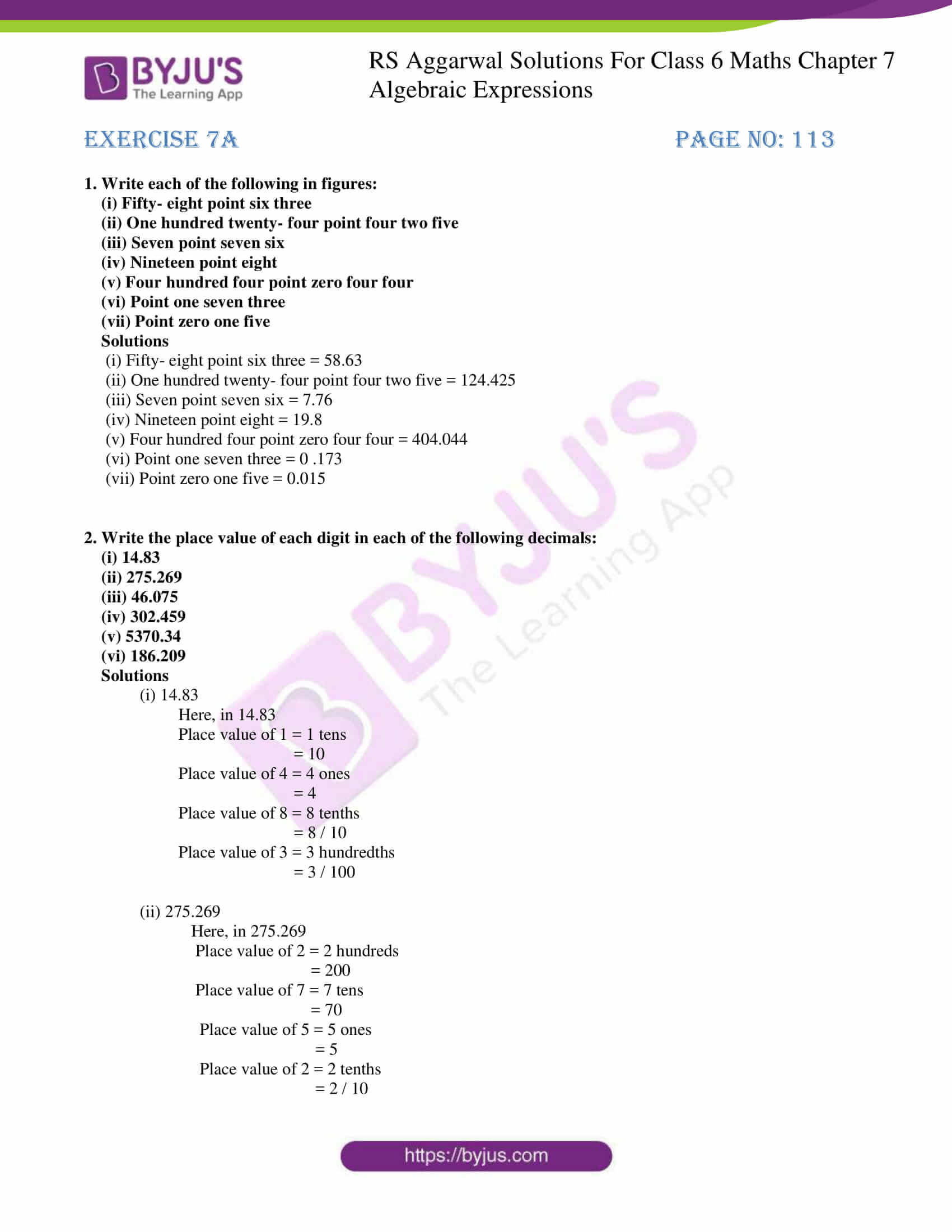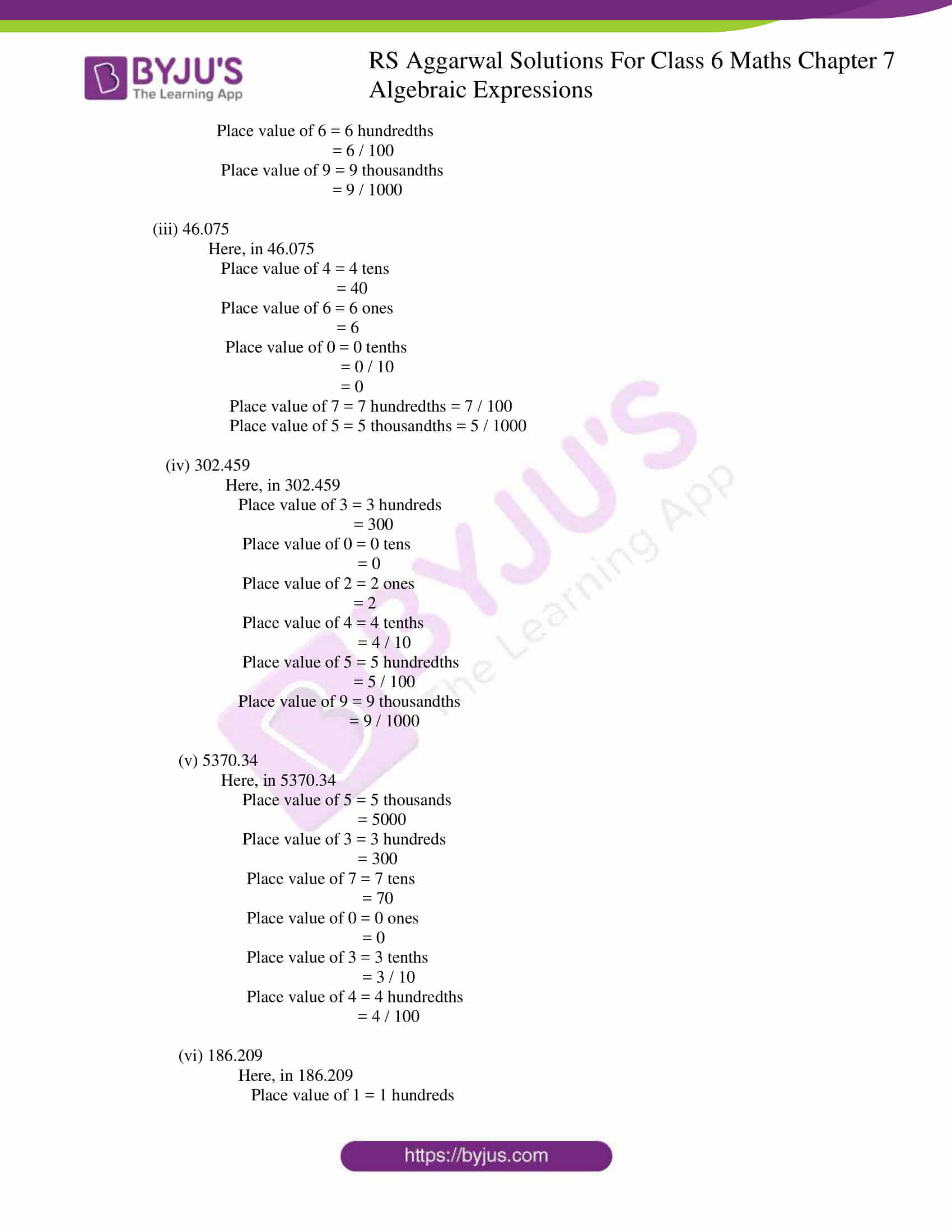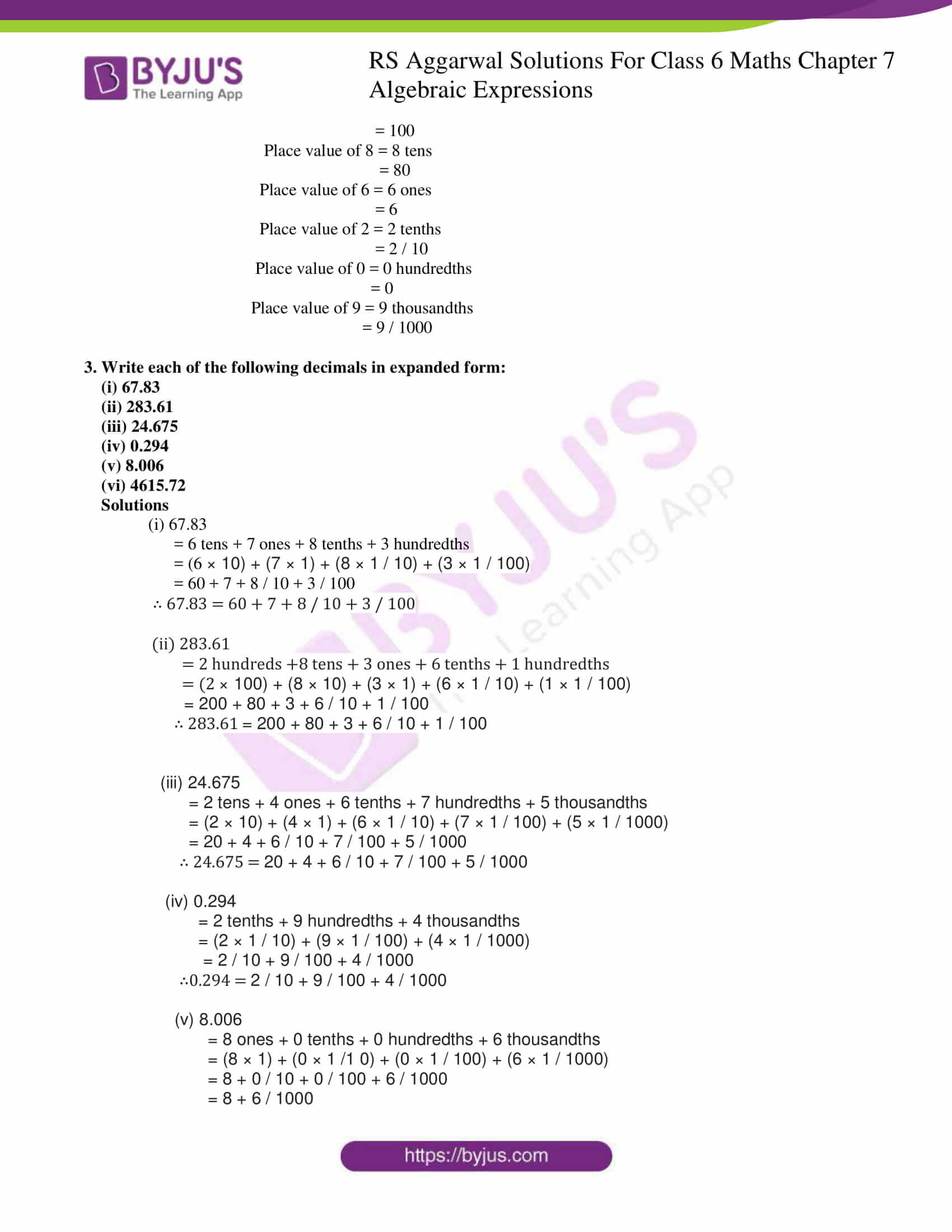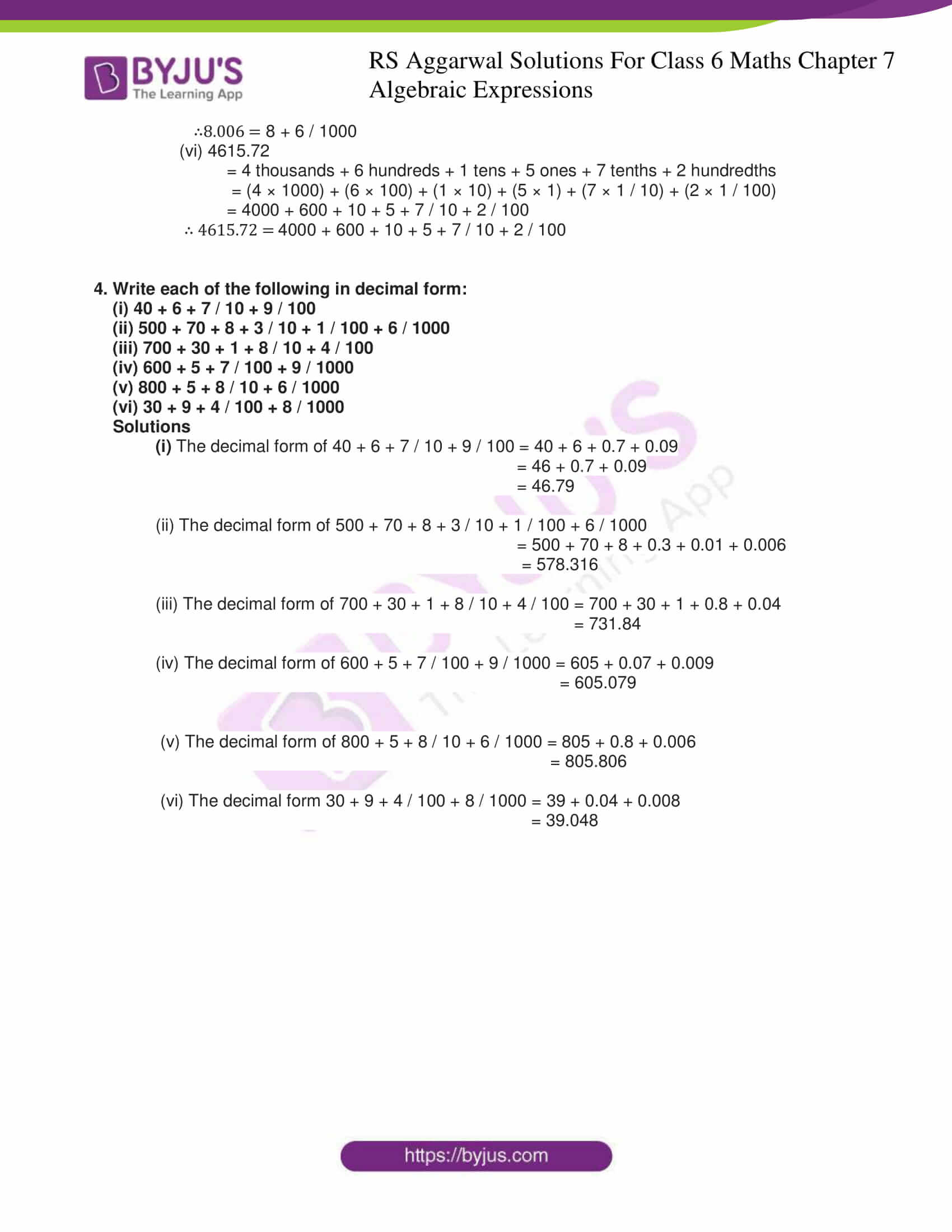# RS Aggarwal Solutions for Class 6 Chapter 7 Decimals Exercise 7A

RS Aggarwal Solutions for Class 6 Chapter 7 Decimals Exercise 7A are provided here for free download. The concepts covered in the first exercise of Chapter 7 are decimal fractions, decimals and types of decimals. The fractions in which the denominators are 10, 100, 1000, etc. are decimal fractions. The numbers which are expressed in decimal form are called decimals. Decimals having the same number of Decimal places are called Like Decimals and Decimals having a different number of Decimal places are called Unlike Decimals. Students can download the PDF and refer them to solve exercise wise problems of RS Aggarwal textbook.

## Download PDF of RS Aggarwal Solutions for Class 6 Chapter 7 Decimals Exercise 7A### Access answers to Maths RS Aggarwal Solutions for Class 6 Chapter 7 Decimals Exercise 7A

1. Write each of the following in figures:

(i) Fifty- eight point six three

(ii) One hundred twenty- four point four two five

(iii) Seven point seven six

(iv) Nineteen point eight

(v) Four hundred four point zero four four

(vi) Point one seven three

(vii) Point zero one five

Solutions

(i) Fifty- eight point six three = 58.63

(ii) One hundred twenty- four point four two five = 124.425

(iii) Seven point seven six = 7.76

(iv) Nineteen point eight = 19.8

(v) Four hundred four point zero four four = 404.044

(vi) Point one seven three = 0 .173

(vii) Point zero one five = 0.015

2. Write the place value of each digit in each of the following decimals:

(i) 14.83

(ii) 275.269

(iii) 46.075

(iv) 302.459

(v) 5370.34

(vi) 186.209

Solutions

(i) 14.83

Here, in 14.83

Place value of 1 = 1 tens

= 10

Place value of 4 = 4 ones

= 4

Place value of 8 = 8 tenths

= 8 / 10

Place value of 3 = 3 hundredths

= 3 / 100

(ii) 275.269

Here, in 275.269

Place value of 2 = 2 hundreds

= 200

Place value of 7 = 7 tens

= 70

Place value of 5 = 5 ones

= 5

Place value of 2 = 2 tenths

= 2 / 10

Place value of 6 = 6 hundredths

= 6 / 100

Place value of 9 = 9 thousandths

= 9 / 1000

(iii) 46.075

Here, in 46.075

Place value of 4 = 4 tens

= 40

Place value of 6 = 6 ones

= 6

Place value of 0 = 0 tenths

= 0 / 10

= 0

Place value of 7 = 7 hundredths = 7 / 100

Place value of 5 = 5 thousandths = 5 / 1000

(iv) 302.459

Here, in 302.459

Place value of 3 = 3 hundreds

= 300

Place value of 0 = 0 tens

= 0

Place value of 2 = 2 ones

= 2

Place value of 4 = 4 tenths

= 4 / 10

Place value of 5 = 5 hundredths

= 5 / 100

Place value of 9 = 9 thousandths

= 9 / 1000

(v) 5370.34

Here, in 5370.34

Place value of 5 = 5 thousands

= 5000

Place value of 3 = 3 hundreds

= 300

Place value of 7 = 7 tens

= 70

Place value of 0 = 0 ones

= 0

Place value of 3 = 3 tenths

= 3 / 10

Place value of 4 = 4 hundredths

= 4 / 100

(vi) 186.209

Here, in 186.209

Place value of 1 = 1 hundreds

= 100

Place value of 8 = 8 tens

= 80

Place value of 6 = 6 ones

= 6

Place value of 2 = 2 tenths

= 2 / 10

Place value of 0 = 0 hundredths

= 0

Place value of 9 = 9 thousandths

= 9 / 1000

3. Write each of the following decimals in expanded form:

(i) 67.83

(ii) 283.61

(iii) 24.675

(iv) 0.294

(v) 8.006

(vi) 4615.72

Solutions

(i) 67.83

= 6 tens + 7 ones + 8 tenths + 3 hundredths

= (6 × 10) + (7 × 1) + (8 × 1 / 10) + (3 × 1 / 100)

= 60 + 7 + 8 / 10 + 3 / 100

∴ 67.83 = 60 + 7 + 8 / 10 + 3 / 100

(ii) 283.61

= 2 hundreds +8 tens + 3 ones + 6 tenths + 1 hundredths

= (2 × 100) + (8 × 10) + (3 × 1) + (6 × 1 / 10) + (1 × 1 / 100)

= 200 + 80 + 3 + 6 / 10 + 1 / 100

∴ 283.61 = 200 + 80 + 3 + 6 / 10 + 1 / 100

(iii) 24.675

= 2 tens + 4 ones + 6 tenths + 7 hundredths + 5 thousandths

= (2 × 10) + (4 × 1) + (6 × 1 / 10) + (7 × 1 / 100) + (5 × 1 / 1000)

= 20 + 4 + 6 / 10 + 7 / 100 + 5 / 1000

∴ 24.675 = 20 + 4 + 6 / 10 + 7 / 100 + 5 / 1000

(iv) 0.294

= 2 tenths + 9 hundredths + 4 thousandths

= (2 × 1 / 10) + (9 × 1 / 100) + (4 × 1 / 1000)

= 2 / 10 + 9 / 100 + 4 / 1000

∴0.294 = 2 / 10 + 9 / 100 + 4 / 1000

(v) 8.006

= 8 ones + 0 tenths + 0 hundredths + 6 thousandths

= (8 × 1) + (0 × 1 /1 0) + (0 × 1 / 100) + (6 × 1 / 1000)

= 8 + 0 / 10 + 0 / 100 + 6 / 1000

= 8 + 6 / 1000

∴8.006 = 8 + 6 / 1000

(vi) 4615.72

= 4 thousands + 6 hundreds + 1 tens + 5 ones + 7 tenths + 2 hundredths

= (4 × 1000) + (6 × 100) + (1 × 10) + (5 × 1) + (7 × 1 / 10) + (2 × 1 / 100)

= 4000 + 600 + 10 + 5 + 7 / 10 + 2 / 100

∴ 4615.72 = 4000 + 600 + 10 + 5 + 7 / 10 + 2 / 100

4. Write each of the following in decimal form:

(i) 40 + 6 + 7 / 10 + 9 / 100

(ii) 500 + 70 + 8 + 3 / 10 + 1 / 100 + 6 / 1000

(iii) 700 + 30 + 1 + 8 / 10 + 4 / 100

(iv) 600 + 5 + 7 / 100 + 9 / 1000

(v) 800 + 5 + 8 / 10 + 6 / 1000

(vi) 30 + 9 + 4 / 100 + 8 / 1000

Solutions

(i) The decimal form of 40 + 6 + 7 / 10 + 9 / 100 = 40 + 6 + 0.7 + 0.09

= 46 + 0.7 + 0.09

= 46.79

(ii) The decimal form of 500 + 70 + 8 + 3 / 10 + 1 / 100 + 6 / 1000

= 500 + 70 + 8 + 0.3 + 0.01 + 0.006

= 578.316

(iii) The decimal form of 700 + 30 + 1 + 8 / 10 + 4 / 100 = 700 + 30 + 1 + 0.8 + 0.04

= 731.84

(iv) The decimal form of 600 + 5 + 7 / 100 + 9 / 1000 = 605 + 0.07 + 0.009

= 605.079

(v) The decimal form of 800 + 5 + 8 / 10 + 6 / 1000 = 805 + 0.8 + 0.006

= 805.806

(vi) The decimal form 30 + 9 + 4 / 100 + 8 / 1000 = 39 + 0.04 + 0.008

= 39.048